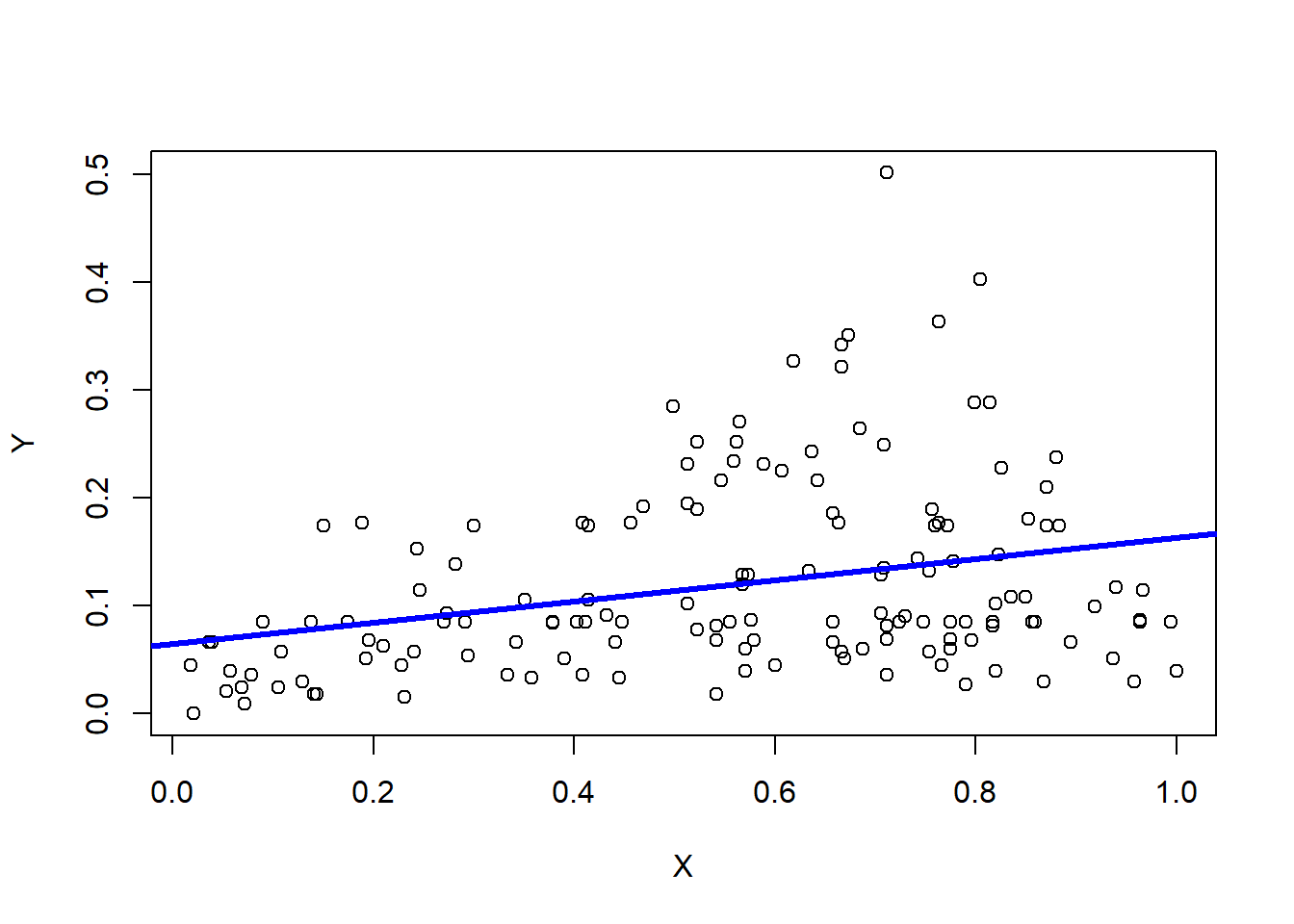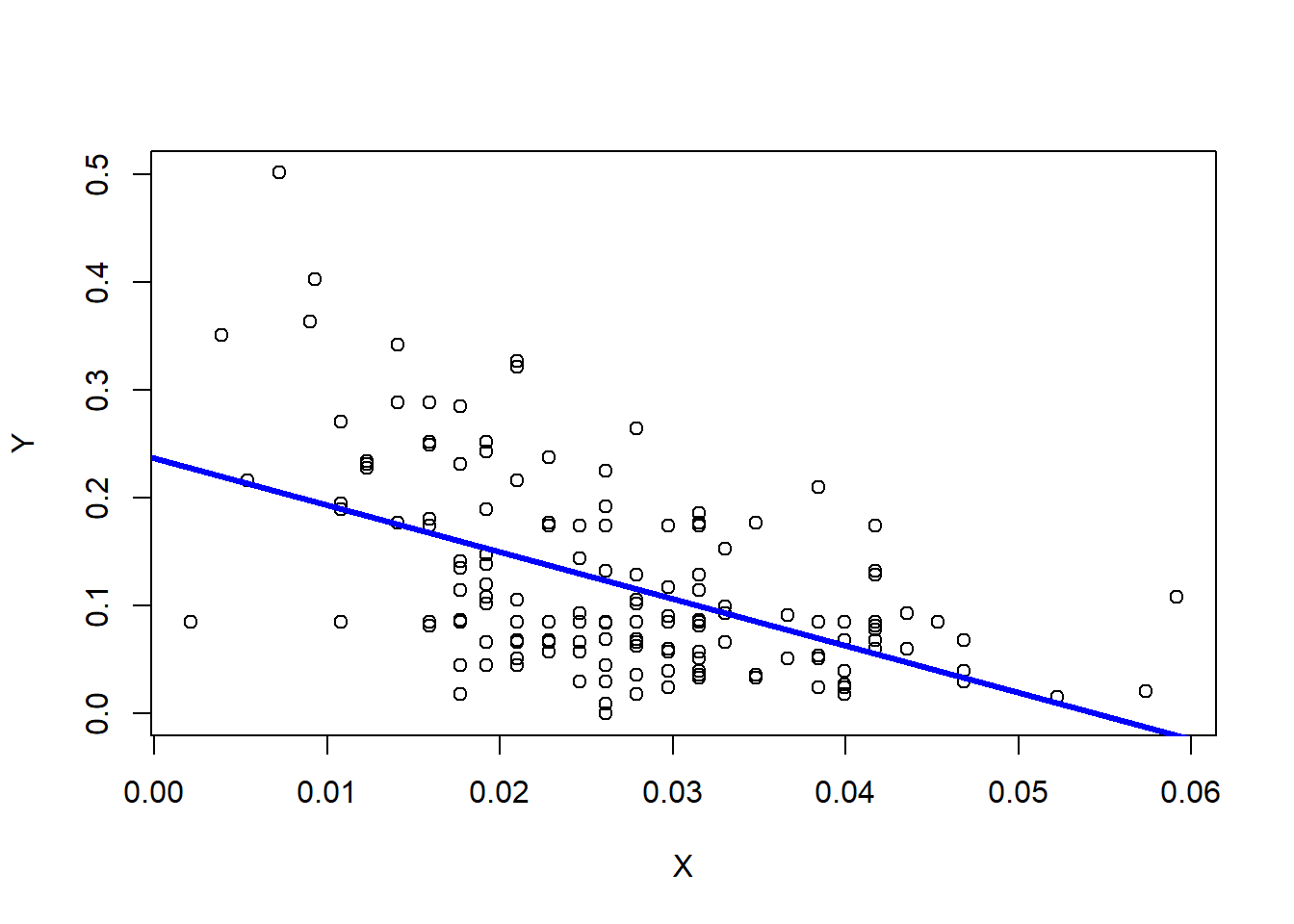Linear Regression establishes a relationship between a Dependent variable i.e. Y and one or more Independent variables i.e X, using a best fit straight line known as Regression Line. The equation of this regresiion line can then be used to predict value of ‘Y’ for any given ‘X’.

        Dependent Variable  (Target)      : Continuous
Independent Variable(Predictor(s)): Continuous/Discrete


Simple linear regression involves one target(Y) and one predictor(X). This demo performs simple linear regression using Least Sqaures Method to find regression line that shows trend in the data i.e. relationship between X and Y . The equation of regression line in slope-intercept form is:

        Y = mX + c   ,where m= slope of straight line
c= Y-intercept


### 1. Load and view dataset

require("datasets")
data("airquality")
str(airquality)
## 'data.frame':    153 obs. of  6 variables:
##  $Ozone : int 41 36 12 18 NA 28 23 19 8 NA ... ##$ Solar.R: int  190 118 149 313 NA NA 299 99 19 194 ...
##  $Wind : num 7.4 8 12.6 11.5 14.3 14.9 8.6 13.8 20.1 8.6 ... ##$ Temp   : int  67 72 74 62 56 66 65 59 61 69 ...
##  $Month : int 5 5 5 5 5 5 5 5 5 5 ... ##$ Day    : int  1 2 3 4 5 6 7 8 9 10 ...
head(airquality)
##   Ozone Solar.R Wind Temp Month Day
## 1    41     190  7.4   67     5   1
## 2    36     118  8.0   72     5   2
## 3    12     149 12.6   74     5   3
## 4    18     313 11.5   62     5   4
## 5    NA      NA 14.3   56     5   5
## 6    28      NA 14.9   66     5   6

### 2. Preprocess the dataset

Let’s begin by finding which attributes have missing values. We then need to impute those missing values(NA), which we will be doing simply by replacing NA with monthly average. Let’s begin!

col1<- mapply(anyNA,airquality) # apply function anyNA() on all columns of airquality dataset
col1
##   Ozone Solar.R    Wind    Temp   Month     Day
##    TRUE    TRUE   FALSE   FALSE   FALSE   FALSE

The output shows that only Ozone and Solar.R attributes have NA i.e. some missing value.

# Impute monthly mean in Ozone
for (i in 1:nrow(airquality)){
if(is.na(airquality[i,"Ozone"])){
airquality[i,"Ozone"]<- mean(airquality[which(airquality[,"Month"]==airquality[i,"Month"]),"Ozone"],na.rm = TRUE)
}
# Impute monthly mean in Solar.R
if(is.na(airquality[i,"Solar.R"])){
airquality[i,"Solar.R"]<- mean(airquality[which(airquality[,"Month"]==airquality[i,"Month"]),"Solar.R"],na.rm = TRUE)
}

}
#Normalize the dataset so that no particular attribute has more impact on clustering algorithm than others.
normalize<- function(x){
return((x-min(x))/(max(x)-min(x)))
}
airquality<- normalize(airquality) # replace contents of dataset with normalized values
str(airquality)
## 'data.frame':    153 obs. of  6 variables:
##  $Ozone : num 0.1201 0.1051 0.033 0.0511 0.0679 ... ##$ Solar.R: num  0.568 0.351 0.444 0.937 0.541 ...
##  $Wind : num 0.0192 0.021 0.0348 0.0315 0.0399 ... ##$ Temp   : num  0.198 0.213 0.219 0.183 0.165 ...
##  $Month : num 0.012 0.012 0.012 0.012 0.012 ... ##$ Day    : num  0 0.003 0.00601 0.00901 0.01201 ...

Yay! We have removed missing values from our dataset. We will now perform Linear Regression on our dataset!

### 3. Apply linear regression algorithm using Least Squares Method on “Ozone” and “Solar.R”

Since simple L.R. requires just one target, let’s take “Ozone” attribute as our target(Y) and “Solar.R” attribute as Predictor(X) to find if there exists any kind of relationship between them.

Y<- airquality[,"Ozone"] # select Target attribute
X<- airquality[,"Solar.R"] # select Predictor attribute

model1<- lm(Y~X)
model1 # provides regression line coefficients i.e. slope and y-intercept
##
## Call:
## lm(formula = Y ~ X)
##
## Coefficients:
## (Intercept)            X
##     0.06509      0.09849
plot(Y~X) # scatter plot between X and Y
abline(model1, col="blue", lwd=3) # add regression line to scatter plot to see relationship between X and YThe above graph shows that slope of the line goes upwards, hence, there exists a positive correlation between ‘Ozone’ and ‘Solar.R’. So, if we increase X, the value of Y will also increase and vice-versa.

### 4. Apply linear regression algorithm using Least Squares Method on “Ozone” and “Wind”

We will perform linear regression to find relationship of “Ozone” with “Wind” now.

Y<- airquality[,"Ozone"] # select Target attribute
X<- airquality[,"Wind"] # select Predictor attribute

model2<- lm(Y~X)
model2 # provides regression line coefficients i.e. slope and y-intercept
##
## Call:
## lm(formula = Y ~ X)
##
## Coefficients:
## (Intercept)            X
##      0.2364      -4.3410
plot(Y~X) # scatter plot between X and Y
abline(model2, col="blue", lwd=3) # add regression line to scatter plot to see relationship between X and YThe above graph shows that slope of the line goes downwards, hence, there exists a negative correlation between ‘Ozone’ and ‘Wind’. So, if we increase X, the value of Y will decrease and vice-versa.

From the above 2 graphs we can conclude that “Solar.R” is positively related to “Ozone” whereas “Wind” is negatively related.

### 4. Perform prediction

Now, let’s use the line coefficients for two equations that we got in model1 and model2 to predict value of Target for any given value of Predictor.

# Prediction of 'Ozone' when 'Solar.R'= 10
p1<- predict(model1,data.frame("X"=10))
p1
##        1
## 1.049993

The predicted value of “Ozone” is 1.0499933 when “Solar.R”= 10

# Prediction of 'Ozone' when 'Wind'= 5
p2<- predict(model2,data.frame("X"=5))
p2
##         1
## -21.46849

The predicted value of “Ozone” is -21.4684949 when “Wind”= 5

You may also wish to try out Data Classification, Clustering or Linear Regression from following links:

1. k-NN Classification for beginners

Using Iris Dataset

Using Airquality Dataset
2. k-means Clustering for beginners

Using Iris Dataset

Using Airquality Dataset
3. Linear Regression for beginners

Using Iris Dataset

Good luck! :)